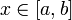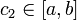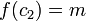# Extreme value theorem

Jump to: navigation, search

## Definition

Suppose$f$ is a continuous function on a closed interval$[a,b]$ (note that$f$ may be defined on a bigger domain, but we are interested in the restriction of$f$ to the closed interval and require it to be continuous). Then,$f$ attains its minimum and maximum value on the interval. In other words, the following statements are true:

1. Existence of maximum value: There is a real number$M$ such that$f(x) \le M$ for all$x \in [a,b]$ and there exists$c_1 \in [a,b]$ such that$f(c_1) = M$.
2. Existence of minimum value: There is a real number$m$ such that$f(x) \ge m$ for all$x \in [a,b]$ and there exists$c_2 \in [a,b]$ such that$f(c_2) = m$.